Mobile QR Code1. (Department of MSDE, Seoul National University of Science and Technology, Seoul, Korea )

Low temperature, high magnetic field, PN junction diodes, Schottky barrier diodes, ideality factor, magnetoresistance, CMOS

## I. INTRODUCTION

Recently, the interest in the operation of CMOS circuits under a cryogenic environment is growing for the realization of quantum computers with higher qubits (quantum bits) (1,2). The monolithic integration of the read-out and control circuitry in CMOS would resolve the interface complexity to enable practical quantum computers with enhanced compactness and reliability. A qubit for topological quantum computing using FQHE (Fractional Quantum Hall Effect) requires a high magnetic field as well as a cryogenic environment (3). It is necessary to investigate the behaviors of CMOS devices under a high magnetic field as well as cryogenic temperature in this case.

An EPR (Electron Paramagnetic Resonance) spectroscopy should be another application using cryogenic temperature and high magnetic field environment (4). It is one of the most popular techniques with a wide range of applications in chemistry, physics, biology and medicine. In EPR spectroscopy, samples are often cooled down to a cryogenic temperature under a high magnetic field to get stable spectra and study their temperature dependence. Therefore, EPR spectroscopy employs a special cryostat with a superconducting magnet. Long rigid waveguides are often used to deliver RF signal into a sample cavity inside the cryostat (4). A considerable transmission loss is unavoidable due to the long waveguide connecting the sample chamber in the magnetic field center and external measurement instruments. If the external instruments can be miniaturized using CMOS technology and deployed near a sample, the EPR sensitivity would be significantly enhanced by reducing the transmission loss inside the cryostat. Indeed some on-chip CMOS spectrometers operated in the room temperature has been reported (5-7).

A junction diode is a basic building block of various CMOS circuits. PN junction diodes (PND) in CMOS have been widely employed in CMOS. Two Schottky barrier junction diodes (SBD) in CMOS, Shallow Trench Separated-SBD (STS-SBD) and Poly Gate Separated-SBD (PGS-SBD), have been introduced relatively recently (8). The Schottky junction of the STS- and PGS- SBD is implemented (separated) using STI (Shallow Trench Insulator) and poly gate, respectively (Fig. 1). Since the SBDs have a good high-frequency performance with the cutoff frequency above 1 THz, various CMOS circuits using the SBDs have been developed (9-12). Yet the characteristics of the CMOS diodes has not been investigated under a cryogenic temperature and high magnetic field environment.

In this paper, DC behaviors of three diode types available in CMOS are characterized under a low temperature and high magnetic field to examine the feasibility of CMOS diode circuits operating in an extreme environment. The temperature dependence of the devices was measured at the temperatures of 300 K, 150 K, 77 K and 4.2 K. To understand the field dependence of the CMOS diodes, measurements were also performed under magnetic fields of 0 T, 2 T, 4 T and 6 T.

Section II describes the test structures and Section III shows the measurement setup to implement the cryogenic and high magnetic field environment. Section IV and V describes the measurement results and conclusion, respectively.

## II. Test Structures

Fig. 1 shows the unit cell cross-section of three diodes: PND, STS-SBD and PGS-SBD (8,13). The diodes with the different configuration are implemented in a 90-nm foundry CMOS technology without any process modifications. The PND is formed in an n-well region (p+/n/n+). The Schottky contacts are formed on diffusion regions where there are no source/drain implants. An ohmic contact placed on an n+ implanted n-well region forms a cathode. The cathode and anode of STS- and PGS-SBD is separated by a shallow trench and polysilicon gate ring, respectively. A vertical current is dominant around the junction of STS-SBD (Fig. 1(b)) while horizontal current is dominant in PGS-SBD (Fig. 1(c)) around the region. The size and number of unit cells are summarized in Table 1 The diodes are comprised of multiple unit cells to achieve a high cutoff frequency (8,13).

Fig. 1. Unit cell cross-section of (a) PND, (b) STS-SBD, (c) PGS-SBD.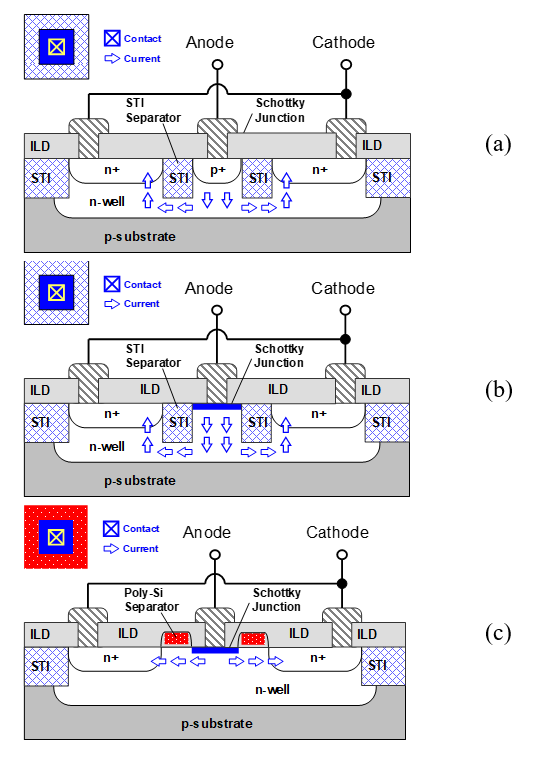Table 1. Summary of diode test structures

 Test structure Unit Cell Area [µ$m^{2}$] Number of cells Area [µ$m^{2}$] PND (p+/n/n+) 0.4 × 0.4 12 1.92 STS-SBD 0.29 × 0.29 16 1.30 PGS-SBD 0.28 × 0.28 16 1.25

## III. Measurement Setup

The schematic and photos of the measurement setup are shown in Fig. 2. The Quantum Design PPMS (Physical Property Measurement System) provides the low-temperature and high-magnetic environment for the measurements (4,14). A test chip is mounted on a sample holder and is put into a sample chamber, which is controlled to a target temperature by regulating helium gas flows surrounding the chamber. J-V characteristics of devices were measured using a semiconductor parameter analyzer (HP 4155A).

Fig. 2. (a) Schematic, (b) photos of the measurement setup.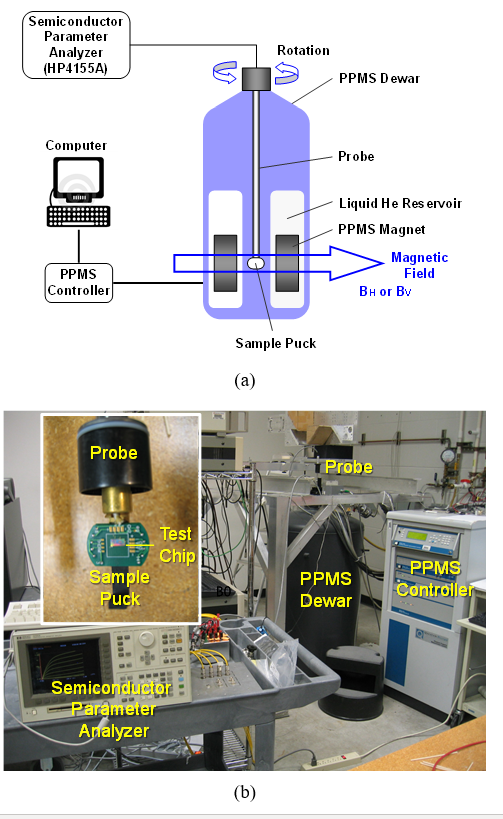To characterize the magnetic field dependence at cryogenic temperatures, the test structures are characterized under the magnetic fields up to 6 T. The measurements are performed for two different field orientations (Fig. 3) by rotating the probe in Fig. 2. The B$_{\mathrm{V}}$ represents the magnetic field perpendicular to the substrate while the B$_{\mathrm{H}}$ represents the magnetic field parallel to the substrate as shown in Fig. 3. A single orientation for B$_{\mathrm{H}}$ is enough since all devices are square-symmetric.

## IV. Measurement Results

### 1. Temperature Dependence

Fig. 4 shows measured J-V curves of the CMOS diodes at the varying temperatures under no magnetic field. V$_{\mathrm{D}}$ and J$_{\mathrm{D}}$ is the applied diode voltage and the diode current density (the ratio of a diode current (I$_{\mathrm{D}}$) to a junction area), respectively. The dominant conduction mechanism of PNDs and SBDs is a diffusion and thermionic emission, respectively (15). A J$_{\mathrm{D}}$ for a fixed V$_{\mathrm{D}}$ in Fig. 4 clearly decreases as the temperature decreases in the PND and SBDs, mainly due to the exponential drop in the carrier concentration and thermionic emission, respectively (15). The SBDs have higher reverse-bias (leakage) current density level like other SBDs while that of PND are smaller than 10$^{\mathrm{-7~}}$mA/µm$^{2}$ (8,13,16). The STS-SBD shows a 10${\times}$ higher leakage current level than the PGS-SBD. In the forward bias region, the diodes are operated in either the low-level injection (LLI) or high-level injection (HLI) region depending on the current density level (17). The reduced J-V slope in the HLI region can be explained by the injection of excess minority carriers (17,18). The PGS-SBD shows a higher forward current level approaching that of the PND in the HLI region.

Fig. 3. Magnetic field orientations for the field dependence measurements of the diodes.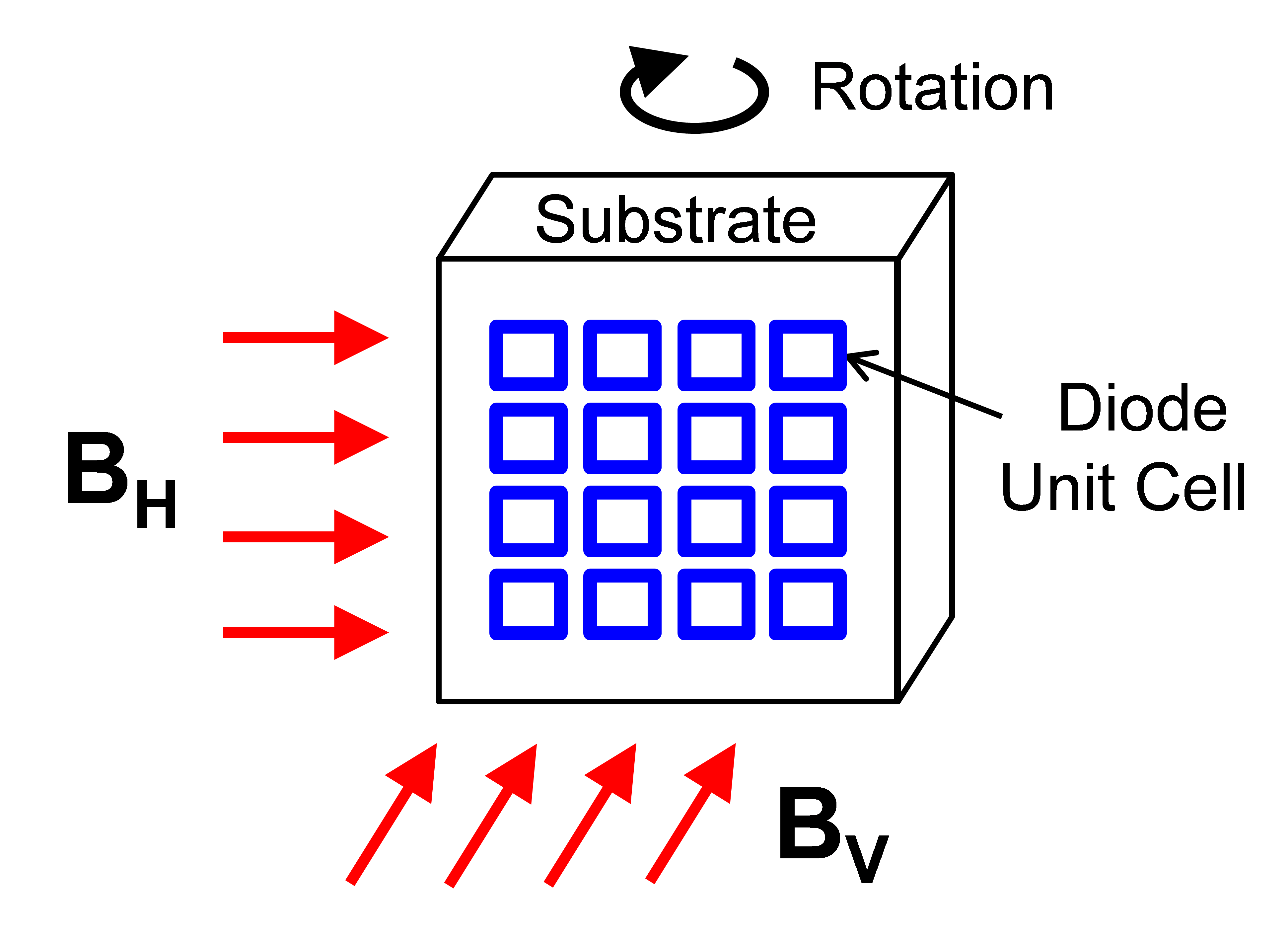Fig. 4. J-V characteristics of (a) PND and STS-SBD, (b) PND and PGS-SBD at varying temperatures.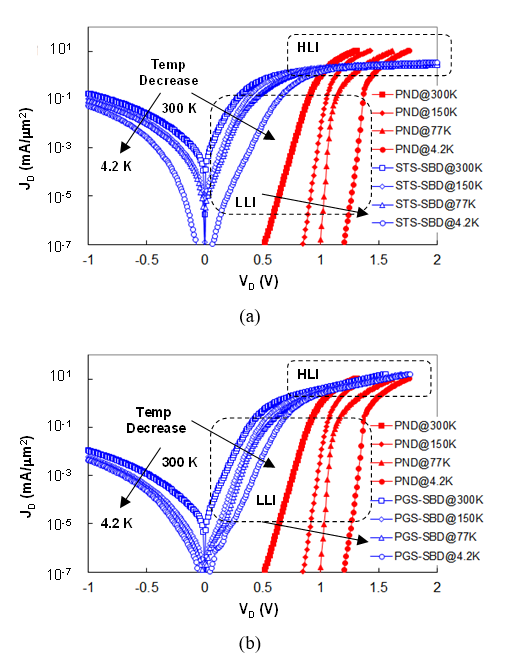Table 2. Measured ideality factor (n) of PND, STS-SBD and PGS-SBD at the varying temperatures

 Temp (K) PND STS-SBD PGS-SBD 300 1.04 1.62 1.42 150 1.04 2.73 2.42 77 1.05 5.44 4.46 4.2 40.5 126 111

Fig. 5. Saturation current density (J$_{\mathrm{S}}$) and Schottky barrier height (Φ$_{\mathrm{B0}}$) of the STS- and PGS-SBD.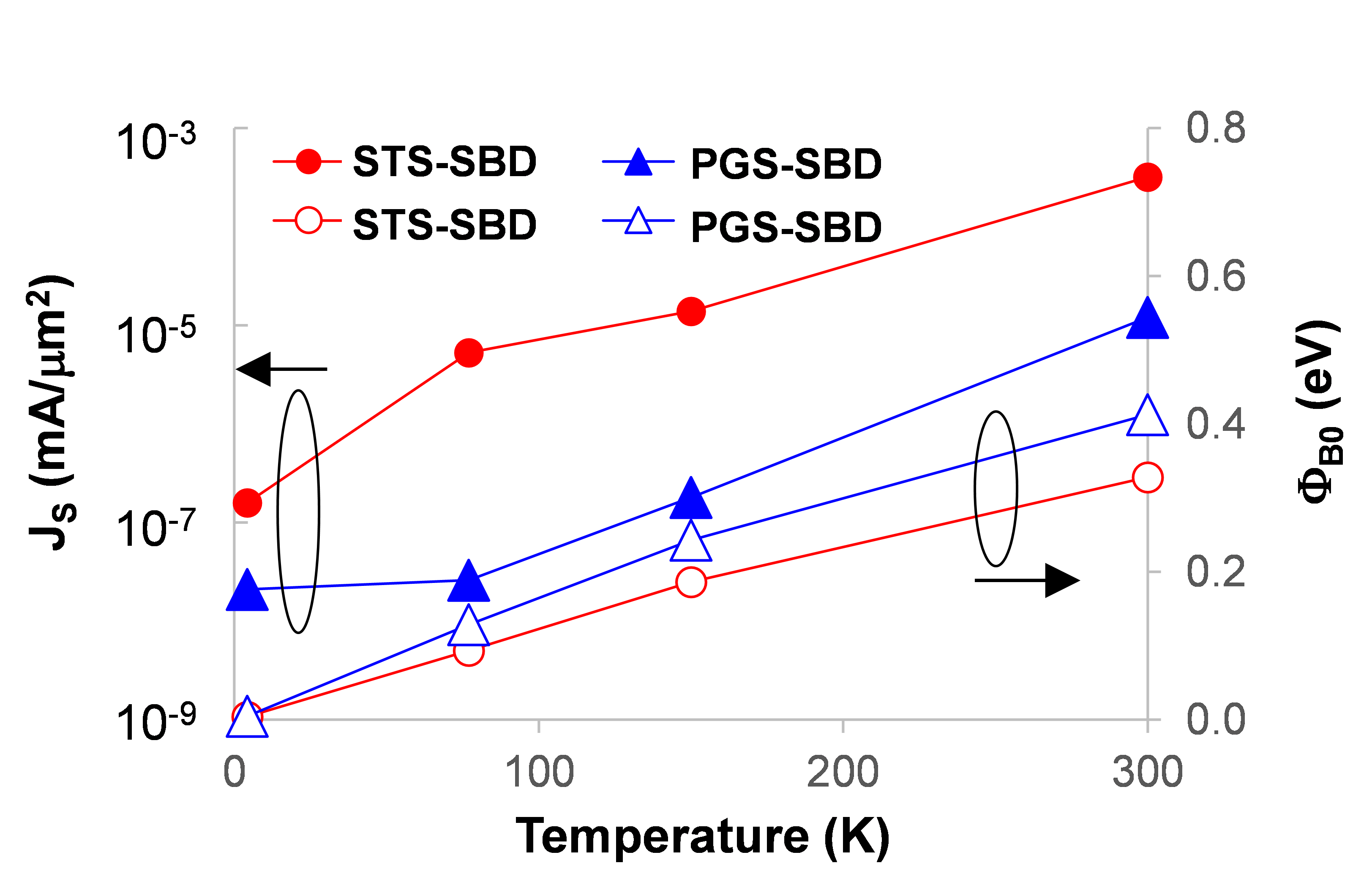The relationship between the V$_{\mathrm{D}}$ and J$_{\mathrm{D}}$ of the PND and SBDs in the LLI regions in Fig. 4 can be approximated by (1)(15).

##### (1)
$\ln \left(J_{D}\right) \approx \ln \left(J_{S}\right)+\left(\frac{q}{n k T}\right) V_{D}$

J$_{\mathrm{S}}$ is the saturation current, q is the electric charge, n is the ideality factor, k is the Boltzmann’s constant, T is the absolute temperature in Kelvin. The n value can be extracted from the slope of the J-V curves in the LLI region. The J$_{\mathrm{S}}$ value can be extracted from the asymptotic straight line intercept at zero bias (16).

The extracted ideality factors (n) are listed in Table 2 The PND (p+/n/n+) shows typical characteristic down to 77 K with an ideality factor close to one. The ideality factor of PND at 4.2 K is 40.5. This is perhaps due to the fact that the forward J-V characteristic of Si p-n junction below 30 K is dominated by the thermionic emission of carriers over a small energy barrier (19). The measured ideality factors of SBDs rapidly increase as the temperature decreases. The temperature dependency has been successfully analyzed based on a thermionic emission mechanism with a spatial distribution of the barrier heights caused by inhomogeneities at the metal-semiconductor interface (16,20-23). Many studies have reported the approximated temperature dependency of n (T) ${\approx}$ 1 + T$_{0}$/T, where T$_{0}$ is a constant independent of temperature (16,21,23). The measurement results in Table. 2 roughly follow the pattern of the relationship. Both SBDs show an abrupt increase in the ideality factor at 4.2 K.

The extracted J$_{\mathrm{S}}$ of SBDs are shown in Fig. 5. The J$_{\mathrm{S}}$ exponentially decreases as the temperature decreases. The J$_{\mathrm{S}}$ of SBDs can be modeled by (2) from the thermionic emission theory (15,16).

##### (2)
$\mathrm{J}_{\mathrm{S}}=\mathrm{A}^{\mathrm{*}}\mathrm{T}^{2}\cdot \exp \left(- \frac{\mathrm{q}Φ _{\mathrm{B}0}}{\mathrm{kT}}\right)$

where A$^{\mathrm{*}}$ is the effective Richardson constant and Φ$_{\mathrm{B0 }}$is the zero-bias Schottky barrier height (15,16). The thermionic emission current of SBDs (J$_{\mathrm{D}}$) is the flow of charge carriers with an enough thermal energy to overcome an energy barrier, and it is given by (3) from (1).

##### (3)
$\mathrm{J}_{\mathrm{D}}\mathit{\approx }\mathrm{A}^{\mathrm{*}}\mathrm{T}^{2}\cdot \exp \left[\frac{- q\left(Φ _{\mathrm{B}0}- \mathrm{V}_{\mathrm{D}}/\mathrm{n}\right)}{\mathrm{kT}}\right]$

Both J$_{\mathrm{S}}$ from (2) and J$_{\mathrm{D}}$ from (3) decrease exponentially as the temperature (T) decreases in the LLI region (Φ$_{\mathrm{B0}}$ > V$_{\mathrm{D}}$), which is roughly consistent with the measurement results in Fig. 4 and 5, respectively.

To further understand characteristics of the SDBs, Φ$_{\mathrm{B0}}$ is calculated from (2)using the obtained J$_{\mathrm{S}}$ (16). A$^{\mathrm{*}}$ of 1.12 µA/µm$^{2}$K$^{2}$ is used for the calculation (24). Fig. 5 plots the calculated Φ$_{\mathrm{B0}}$ for the SBDs. The Φ$_{\mathrm{B0}}$ linearly decreases as the temperature decreases, which is consistent with the analyses in (16,20-23). The discussed spatial distribution of barrier heights is responsible for the temperature dependence of the Φ$_{\mathrm{B0}}$ as well as the ideality factor (n) (16,20-23).

Fig. 6. Turn-on voltage of the diodes under varying temperatures with no magnetic field.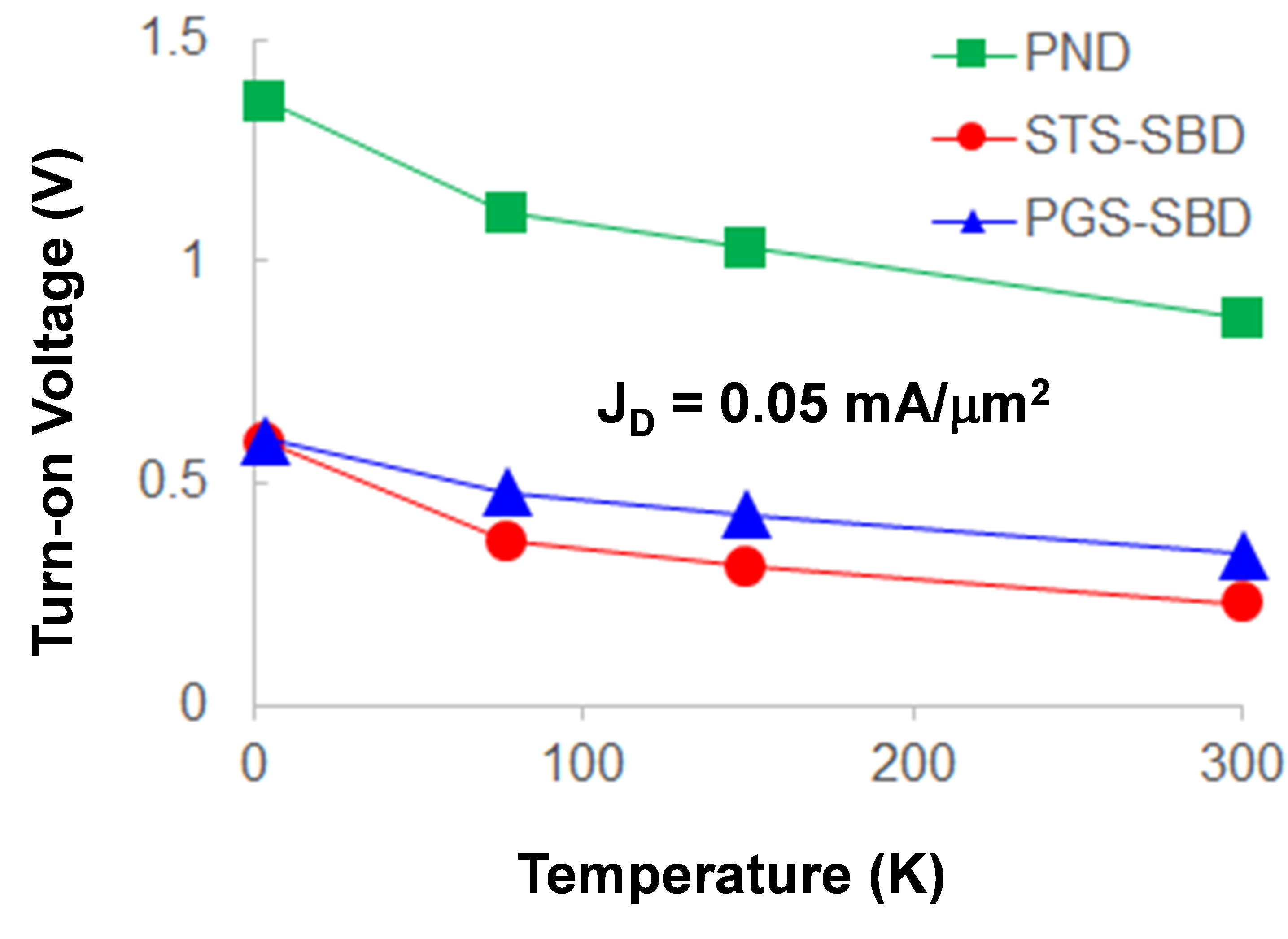Fig. 7. Magnetoresistance (MR) depending on the diode current density at (a) 300 K, (b) 150 K, (c) 77 K, (d) 4.2 K under the magnetic field of 6 T.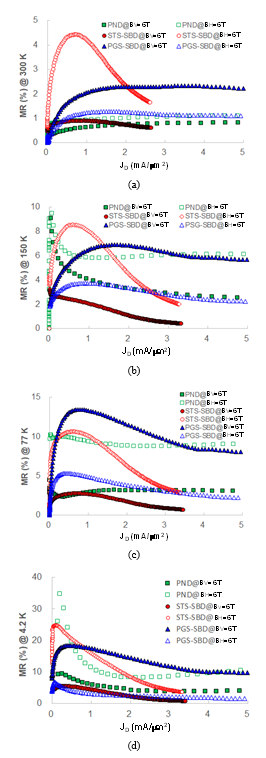The turn-on voltage (at the J$_{\mathrm{D}}$ of 0.05 mA/µm$^{2}$) of the diodes for the different temperatures is extracted and compared (Fig. 6). The turn-on voltages of SBDs are 0.6-0.8 V lower than that of PND as expected. The turn-on voltage of the diodes increases by 0.2-0.5 V as the temperature decreases. The PSG-SBD and STS-SBD have similar values.

### 2. Magnetic Field Dependence

Some materials change their electrical resistivity when an external magnetic field is applied. The phenomenon is called magnetoresistance (MR) effect and MR ratio is given by (4)(25). R(B) and R(0) is the resistance (V$_{\mathrm{D}}$/I$_{\mathrm{D}}$) at the magnetic field density of B and 0 T, respectively.

##### (4)
$\mathrm{MR}\left(\% \right)=\frac{\mathrm{R}\left(\mathrm{B}\right)- \mathrm{R}\left(0\right)}{\mathrm{R}\left(0\right)}\times 100$

Fig. 7 plots the measured MR for various current density level (J$_{\mathrm{D}}$) at the different temperatures and the same magnetic field of 6 T. The MRs saturate or slowly drop as J$_{\mathrm{D}}$ increases. The behavior can be explained by the space-charge effect induced by the injection of a large amount of carriers (26,27). The STS-SBD shows the high peak MRs at the J$_{\mathrm{D}}$ smaller than 1 mA/µm$^{2}$ under the horizonal magnetic field of 6 T. The maximum MR of 35% is observed in the PND under the temperature of 4.2 K. The overall MRs increase as the temperature decreases. The classical magnetoresistance associated with the orbital motion of carriers is proportional to (µB)$^{2}$ where µ is the carrier mobility (25). Due to the lattice vibrations, µ increases as temperature decreases in a semiconductor (15). This would partially explain the temperature dependency of MRs in Fig. 7.

Fig. 8. Magnetoresistance (MR) depending on magnetic fields at (a) 300 K, (b) 150 K, (c) 77 K, (d) 4.2 K in the high-level injection (HLI) region.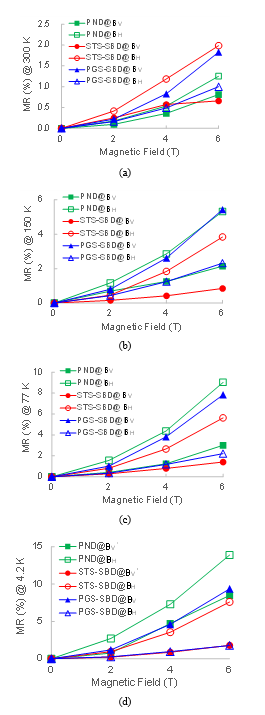Fig. 8 plots MRs for varying magnetic fields in the HIL region at the temperatures. The MRs are measured at J$_{\mathrm{D}}$ of 10.4, 2.3 and 8.0 mA/µm$^{2}$ for the PND, STS-SBD and PGS-SBD, respectively. Curve fittings show that the MRs quadratically increase as the magnetic field increases ($\mathrm{MR}\propto B_{H,V}^{\alpha }$ where ${\alpha}$ = 1.85-1.97), which is consistent with the discussed theoretical estimation, $\mathrm{MR}\propto \left(\mu B\right)^{2}$ (25). Again, the overall MRs increase as the temperature decreases. It is observed that the MR of PND increases faster than others as the temperature decreases. The PND has the maximum magnetoresistance of 13.9% in the HLI region under the temperature of 4.2 K and horizontal magnetic field of 6 T. All measured MRs are positive and no negative MR is observed (28).

The devices show clear dependence on the orientation as well as the strength of the applied magnetic fields. The horizontal field results in higher MRs than the vertical one in PND in Fig. 8. The PND shows 1.5-3.0${\times}$ higher MRs for the horizontal magnetic fields than for the vertical ones at 6 T. The phenomenon may be explained by the asymmetric geometry of the space charge region in p-n junction induced by the horizontal magnetic field (29,30). The current paths are deflected in the vicinity of the space charge region to increase the effective resistance.

The STS-SBD and PGS-SBD shows larger MRs under the horizontal and vertical magnetic field, respectively, as shown in Fig. 8. The STS-SBD shows 3.0-4.5${\times}$ higher MRs for the horizontal magnetic fields at 6 T. The PGS-SBD shows 1.8-5.3${\times}$ higher MRs for the vertical magnetic fields at 6 T. A magnetoresistance occurs when a current flows perpendicular to a magnetic field (25). The vertical current around the junction of STS-SBD (Fig. 2(b)) would results in the higher magnetoresistance under a horizonal magnetic field. The horizontal current around the junction of PGS-SBD (Fig. 2(c)) would results in a higher magnetoresistance under a vertical magnetic field likewise.

## V. CONCLUSIONS

The CMOS diode devices available in a 90-nm CMOS technology are characterized at the cryogenic temperatures and high magnetic field environment. The temperature dependences of diodes were measured at 300, 150, 77 and 4.2 K. The PND showed the expected behavior down to 77 K with an ideality factor close to one. Schottky barrier diodes have a higher ideality factor with decreasing temperature mainly due to the thermionic emission mechanism with a spatial distribution of the barrier heights. Further investigation on the abrupt increase of ideality factors at 4.2 K in the diodes is needed for better explanation. The zero-bias Schottky barrier height and the turn-on voltage decreases and increases, respectively, as the temperature decreases as expected. The magnetic field dependence of the diodes is also characterized at high magnetic fields up to 6 T at 4.2 K. The MR saturates or slowly drops as the diode current density level increases. The overall MRs increase as the temperature decreases and the magnetic field increases. The devices show clear dependence on the orientation as well as the strength of the applied magnetic fields. The larger MR are observed for the PND and STI-SBD have a larger MR for the horizontal orientation while the PGS-SBD has a larger MR for the vertical orientation. Among the three diodes, the PND has the maximum magnetoresistance of 35% and 13.9% in LLI and HLI region, respectively, under the temperature of 4.2 K and horizontal magnetic field of 6T. The measurement results would be useful for the implementation of CMOS diode circuits in the harsh environment for quantum computation or EPR spectroscopy applications.

### ACKNOWLEDGMENTS

This study was supported by the Research Program funded by the SeoulTech (Seoul National University of Science & Technology). The author would like to thank the valuable supports of Dr. Kenneth K. O in University of Texas at Dallas and Dr. Hill in Florida State University.

### REFERENCES

1
Patra B., Incandela R. M., van Dijk J. P. G., Homulle H. A. R., Song L., Shahmohammadi M., Staszewski R. B., Vladimirescu A., Babaie M., Sebastiano F., Charbon E., Sep 2018, Cryo-CMOS Circuits and Systems for Quantum Computing Applications, IEEE Journal of Solid-State Circuits, Vol. 53, No. 1, pp. 1-132
Charbon E., Sebastiano F., Vladimirescu A., Homulle H., Visser S., Song L., Incandela R. M., Dec 2016, Cryo-CMOS for quantum computing, in IEEE Electron Devices Meeting (IEDM), pp. 343-3463
Averin D. V., Goldman V. J., Dec 2001, Quantum computation with quasiparticles of the fractional quantum Hall effect, Solid State Communications, Vol. 121, No. , pp. 25-284
Mola M., Hill S., Goy P., Gross M., Jan 2000, Instrumentation for Millimeter-wave Magneto-electrodynamic Investigations of Low-Dimensional Conductors, Superconductors, Rev. Sci. Inst., Vol. 71, No. 1, pp. 186-2005
Yalcin T., Boero G., 2008, Single-chip detector for electron spin resonance spectroscopy, Review of Sci. Inst., Vol. 79, No. 0941056
Correia J. H., de Graaf G., Bartek M., Wolffenbuttel R. F., Nov 2002, A single-chip CMOS optical microspectrometer with light-to-frequency converter, bus interface, IEEE Journal of Solid-State Circuits, pp. 1344-13477
Hong L., Sengupta K., Dec 2017, Fully Integrated Optical Spectrometer in Visible, Near-IR in CMOS, IEEE Transactions on Biomedical Circuits Systems, Vol. 11, No. 6, pp. 1176-11918
Sankaran S., O K. K., Jul 2005, Schottky Barrier Diodes for mm-Wave, Detection in a Foundry CMOS Process, IEEE Electron Device Letts., Vol. 26, No. 7, pp. 492-4949
Mao C., Nallani C. S., Sankaran S., Seok E., O K. K., May 2009, 125-GHz Diode Frequency Doubler in 0.13-${\mathrm{\mu}}$m CMOS, IEEE J. Solid-State Circuits, Vol. 44, No. 5, pp. 1531-153810
Han R., Zhang Y., Coquillat D., Viderlier H., Kanp W., Brown E., O K. K., Nov 2011, A 280-GHz Schottky Diode Detector in 130-nm Digital CMOS, IEEE J. Solid-State Circuits, Vol. 46, No. 1111
Shim D., O K. K., Jun 2016, Self-biased Anti-parallel Diode Pair in 130-nm CMOS, IEE Electron. Lett., Vol. 52, No. 13, pp. 1147-114912
Shim D., Choi W., Lee J., O K. K., Oct 2017, Self-Dynamic, Static Biasing for Output Power, Efficiency Enhancement of Complementary Antiparallel Diode Pair Freqeuncy Tripler, IEEE Microw. Wireless Compon. Lett., Vol. 27, No. 12, pp. 1110-111213
Sankaran S., Mao C., Seok E., Shim D., Cao C., Han R., Hung C., O K. K., Feb 2009, Towards THz Operation of CMOS, in IEEE ISSCC Dig. Tech. Papers, pp. 202-20315
Sze S. M., 1969, Physics of Semiconductor Devices, Wiley Interscience & Sons16
Joythi I., Yang H., Shim K., Janardhanam V., Kang S., Hong H., Choi C., Sep 2013, Temperature Dependency of Schottky Barrier Parameters of Ti Schottky Contacts to Si-on-Insulator, Material Transactions, Vol. 54, No. 9, pp. 1655-166017
Muller R. S., Kamins T. I., 2002, Device Electronics for Integrated Circuits, Wiley 3$^{\mathrm{rd}}$ ed18
Ng W. T., Liang S., Salama C. A. T., Jan 1990, Schottky Barrier Diode Characteristics under High Level Injection, Solid-State Electronics, Vol. 33, No. 1, pp. 39-4619
Yang Y. N., Coon D. D., Shepard P. F., Oct 1984, Thermionic emission in silicon at temperatures below 30 K, Appl. Phys. Lett., Vol. 45, pp. 752-75420
Song Y. P., Van Meirhaeghe R. L., Laflere W. H., Cardon F., Jun 1986, On the difference in apparent barrier height as obtained from capacitance-voltage, current-voltage-temperature measurements on Al/p-InP Schottky barriers, Solid-State Electronics, Vol. 29, No. 6, pp. 633-63821
Werner J. H., Guttler H. H., Feb 1991, Barrier inhomogeneities at Schottky contacts, Appl. Phys. Lett., Vol. 69, pp. 1522-153322
Gumus A., Turut A., Yalcin N., Jan 2002, Temperature dependent barrier characteristics of CrNiCo alloy Schottky contacts on n-type molecular-beam epitaxy GaAs, Journal of Applied Physics, Vol. 91, No. 1, pp. 245-25023
Hudait M. K., Venkateswarlu P., Krupanidhi S. B., Jan 2001, Electrical transport characteristics of Au/n-GaAs Schottky diodes on n-Ge at low temperatures, Solid-State Electronics, Vol. 45, No. 1, pp. 133-14124
Andrews J. M., Lepselter M. P., 1970, Reverse Current-Voltage Characteristics of Metal-Silicide Schottky Diodes, Solid State Electronics, Vol. 13, pp. 1011-102325
Assen M., 2017, Review of Magnetoresistance, Hall Effect in Metals, Semiconductors, Master thesis Addis Ababa University26
Delmo M., Kasai S., Kobayashi K., Ono T., Sep 2009, Current-controlled magnetoresistance in silicon in non-Ohmic transport regimes, Applied Physics Letter, Vol. 95, No. 13210627
Delmo M. P., Yamamoto S., Kasai S., Ono T., Kobayashi K., Feb 2009, Large positive magnetoresistive effect in silicon induced by the space-charge effect, Nature, Vol. 457, pp. 1112-111528
Borblik V. L., Rudnev I. A., Shawarts Y. M., Shwarts M. M., 2011, Negative magnetoresistance of heavily doped silicon p-n junction, Semiconductor Physics Quantum Electronics & Optoelectronics, Vol. 14, No. 1, pp. 88-9029
Yang D. Z., Wang T., Sui W. B., Si M. S., Guo D. W., Shi Z., Wang F. C., Xue D. S., Sep 2015, Temperature-Dependent Asymmetry of Anisotropic Magnetoresistance in Silicon p-n Junctions, Scientific Reports, Vol. 5, No. 1109630
Wang T., Si M., Yang E., Shi Z., Wang F., Yang Z., Zhou S., Xue D., Jan 2014, Angular dependence of the magnetoresistance effect in a silicon based p-n junction device, Nanoscale, Vol. 6, No. 3978## Author

received the B.S. and M.S. degrees from the Seoul National University, Seoul, Korea, in 1996 and 1998, respectively and Ph.D degree in the University of Florida in 2011.

In 1998, he joined Samsung Advanced Institute of Technology (SAIT), where he mainly worked on the design and development of RF integrated passive devices and circuits for wireless applications.

In 2011, he joined the Faculty of Seoul National University of Science & Technology (SeoulTech), Korea, where he is an associate professor.

His research interests are in the design and implementation of multi-disciplinary engineering applications.